Open in App
Not now

# Piecewise Function

• Last Updated : 21 Feb, 2021

A function is a mathematical object which associates each input with exactly one output. For example: If a function takes on any input and gives the output as 3. It can be represented in mathematical form as f(x) = 3. As another example, let’s take f(x) = x2, this function behaves in the same way for all the values in its domain. But there can also be some functions that behave differently for different parts of the input.

For example: Let f(x) be that function, let’s say if x ∈ (0,3) f(x) = x and for all other x, f(x) = 1.

This function behaved differently for two types of input. These kinds of functions are called piecewise functions. Let’s introduce them more formally. The figure below also describes a piecewise function. Note how the graph of the function changes for different segments of the input.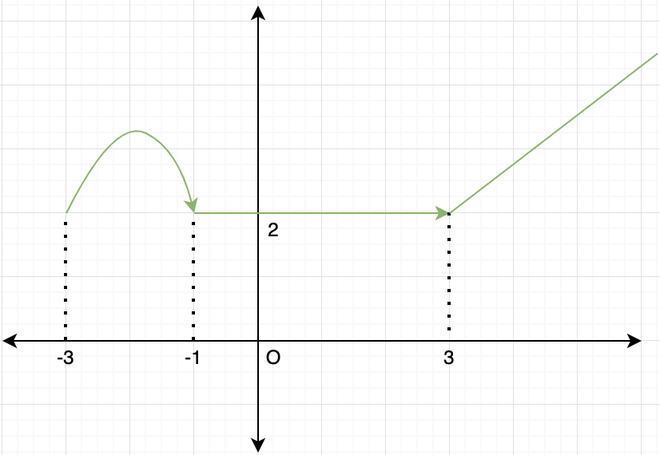An example of a piecewise function.

## Piecewise Functions

A piecewise function is a function that is defined on a sequence of intervals. A classic example of a piecewise function is the absolute value function.

### Absolute Value Function

It has two pieces:

• below zero: -x
• from 0 onwards: x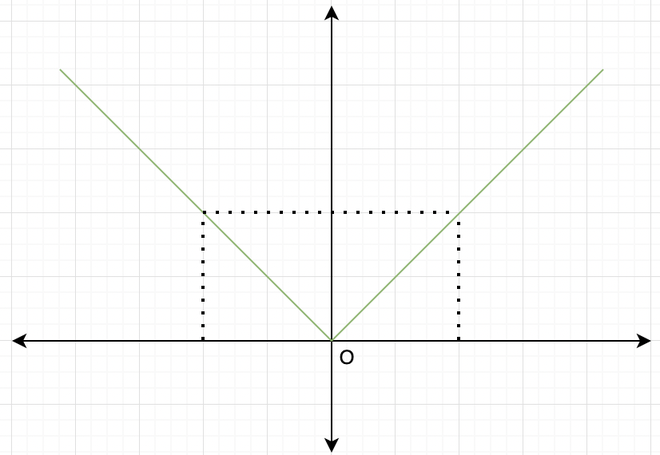Absolute Value Function

### Evaluating Piecewise Functions

Question 1: Find the value of the following function at x = -2,10.Solution:at x = -2, x < 0 so the f(-2) = -2.

at x = 10, x > 0, so f(10) = 10 = 100.

Question 2: An arcade game charges the following prices depending on the length of time:

1. Up to 6 minutes costs Rs.10
2. Over 6 and up to 15 minutes costs Rs.15
3. Over 15 minutes costs Rs.15 plus Rs.1 per minute above 15 minutes

Represent this as a piecewise function and tell the price charged if Anil played the game for 13 minutes and Raju played for 20 minutes.

Solution:

These kind of prices charges can be represented as,at x = 13, f(13) = Rs.15 and x = 20, f(20 ) = 15 + 1( 20 – 15) = 20.

### Step Function

These functions are used a lot in the Electrical and Electronics engineering fields as they are used in Signals and systems. Let’s start with the definition. It is a piecewise function with a finite number of pieces.

Let’s look at this graph,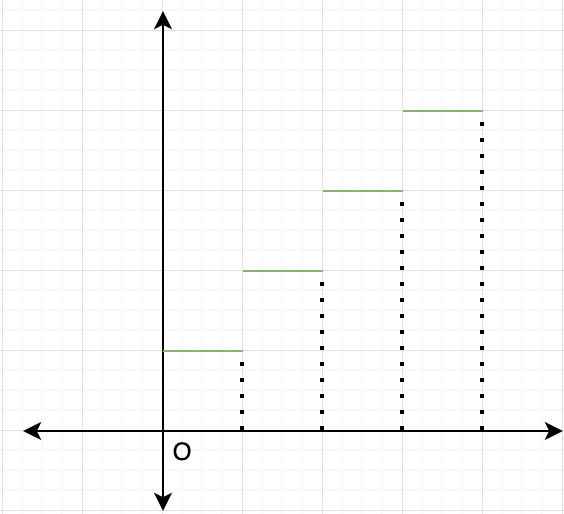The graph looks staircase, they are a class of functions that have a graph that looks similar to a staircase. Two commonly used step functions are floor function and ceiling function.

### Floor Function

The floor function also called the greatest integer function or integer value, gives the largest integer less than or equal to x. The domain for this function is all the real numbers R while the range of this function is all the integers I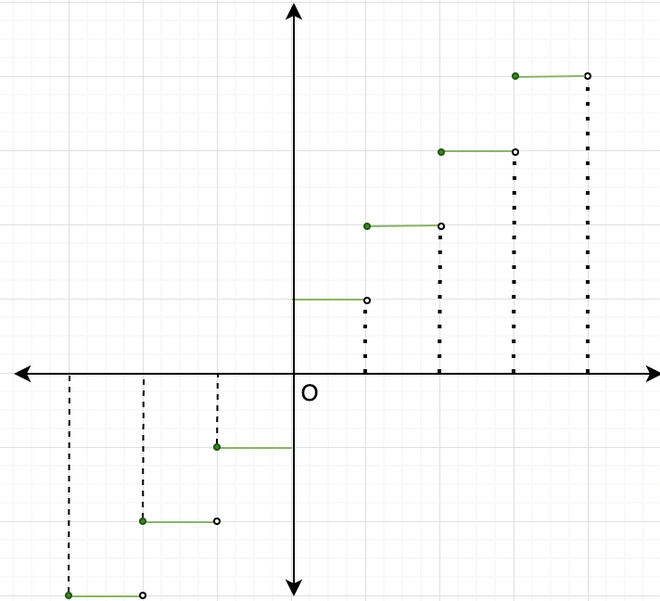Floor Function

Question 1: What is the Floor of 1.43?

Solution:

Floor of a number is the greatest Integer lesser or equal to that number. Therefore, here the Floor of 1.43 is 1.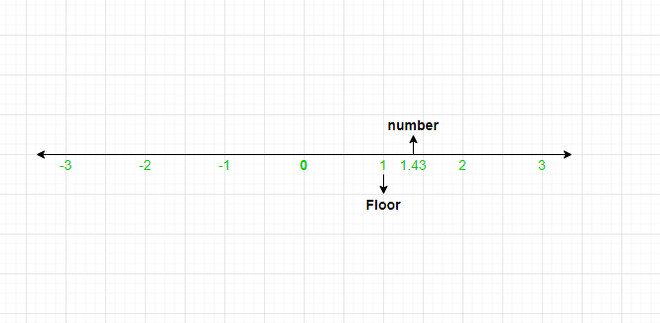Question 2: What is the Floor of -5.66?

Solution:

On Negative axis, the greatest Integer lesser than -5.66 is -6.

Hence, -6 is the Floor of -5.66.

### Ceiling Function

This function returns the smallest successive integer. The ceiling function of a real number x is the least integer that is greater than or equal to the given number x.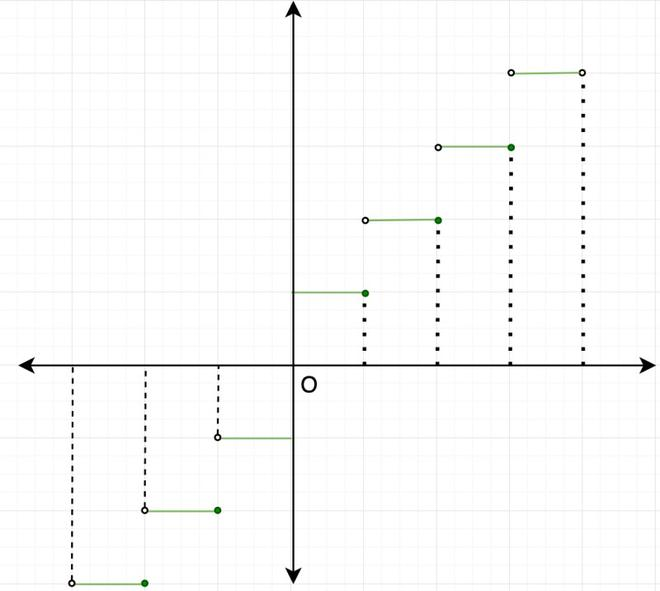Ceiling Function

Similar to floor function, the domain of ceiling function is R and the range is all the integers I.

Question 1: What is the Ceiling of 1.43?

Solution:

The ceiling of the 1.43 should be its smallest successive Integer, hence the ceiling of 1.43 is 2.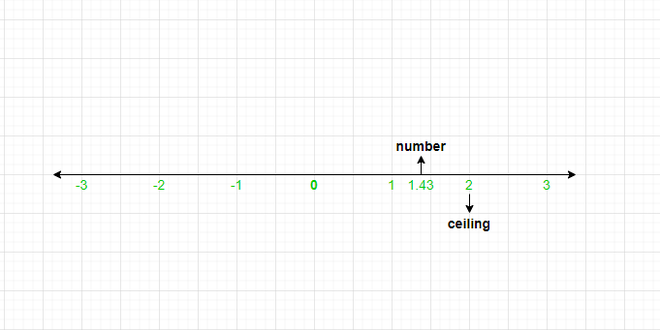Question 2: What is the ceiling of -7.8?

Solution:

The smallest successive Integer of -7.8 is -7.

Hence, -7 is the ceiling of -7.8.

### Unit Step Function

This is yet another type of function used a lot in Signals and systems studies. It is defined as,This function has no value at x = 0. It is called step function because at t = 0, it takes a step from 0 to 1. The domain for this function is R – {0} and range {0,1}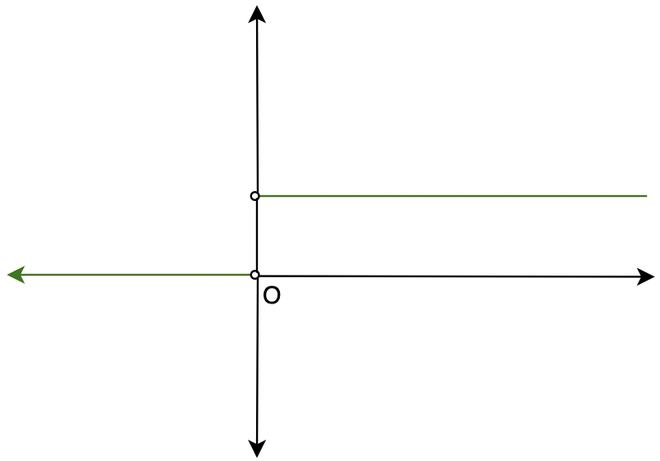Unit Step Function

Question 1: What is the floor of 2.31?

Solution:

Since floor function outputs the nearest smallest integer. floor(2.31) = 2.

Question 2: What is the ceiling value of -4.16?

Solution:

Since the ceiling function returns smallest successive integer, ceil(-4.16) = -4.

Question 3: What is Floor and ceiling of 7?

Solution:

In this case, both the Floor and ceiling of 7 is 7 itself, since it is the largest Integer lesser than or equal to 7 as well as the smallest successive integer of 7.

My Personal Notes arrow_drop_up
Related Articles